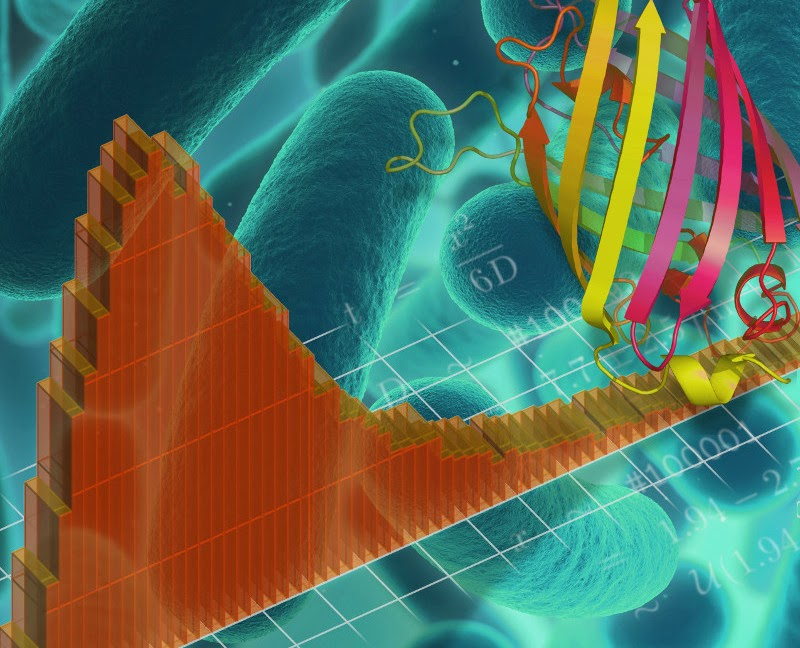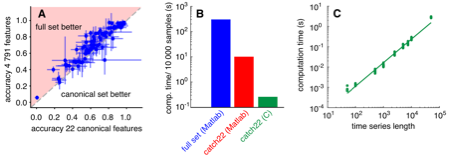## Thursday, 11 December 2014

### Turbocharging the back of the envelope

The numbers that we use to describe the world are rarely exact. How long will it take you to drive to work? Perhaps "between 20 and 30 minutes". It would be unwise (and unnecessary) to say "exactly 23.4 minutes".

This uncertainty means that "back-of-the-envelope" calculations are very valuable in estimating and reasoning about numerical problems, particularly in the sciences. The idea here is to perform a calculation using rough guesses of the quantities involved, to get an "order of magnitude" estimate of the answer you're after. Made famous in physics as "Fermi problems", attributed to Enrico Fermi (who used rough reasoning to deduce quantities from the power of an atomic bomb to the number of piano tuners in Chicago), this approach is integral in many current applications of maths and science. Cool books like "Street-fighting Mathematics", "Guesstimation", "Back of the envelope physics", the excellent "What If?" section of xkcd, and the lateral interview questions facing some job candidates: "how much of the world's water is contained in a cow?" are all examples.Calculations in biology, such as the time it takes for a protein (foreground) to diffuse through an E. coli cell (background), are often subject to large uncertainties. Our approach and web tool allows us to track this uncertainty and obtain a probability distribution over possible answers (plotted).
We've built a free online calculator (Caladis -- calculate a distribution) that complements this approach by allowing one to take the uncertainty in one's estimates into account throughout a calculation. For example, what volume of CO2 is produced by our yearly driving? We could say that we cover 8000 miles per year "give or take" 1000 miles, and find that our car's CO2 emissions are between 100 and 150 grams per kilometre. Our calculator allows us to do the necessary conversions and sums while taking this possible variability into account -- doing maths with "probability distributions" describing our uncertainty. We no longer obtain a single (possibly inaccurate) answer, but a distribution telling us how likely any particular answer is -- in this case a rather concerning bell-shaped distribution between 1 and 2 tonnes which can be viewed here

In the sciences, particularly in biology, measurements often have substantial uncertainties -- due to experimental error, natural variability in the system of interest, or both -- and so using distributions rather than single numbers in calculations allows us to understand and process more about the question of interest. "Back-of-the-envelope" calculations are certainly useful in biology but, owing to the uncertainties involved, one can trust one's estimates better if one has a smart envelope that takes that uncertainty into account.  We've written an accompanying paper "Explicit tracking of uncertainty increases the power of quantitative rule-of-thumb reasoning in cell biology" (free to all in Biophysical Journal) showing how to use our calculator -- in conjunction with the excellent Bionumbers online database, a collection of (often uncertain) experimental measurements in biology -- to make real biological calculations more powerful. Do have a go at using our calculator at www.caladis.org : it's user-friendly and there are lots of examples showing how it works! Iain and Nick